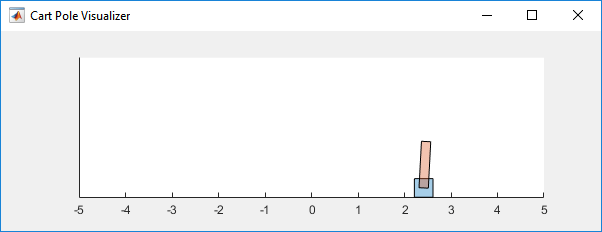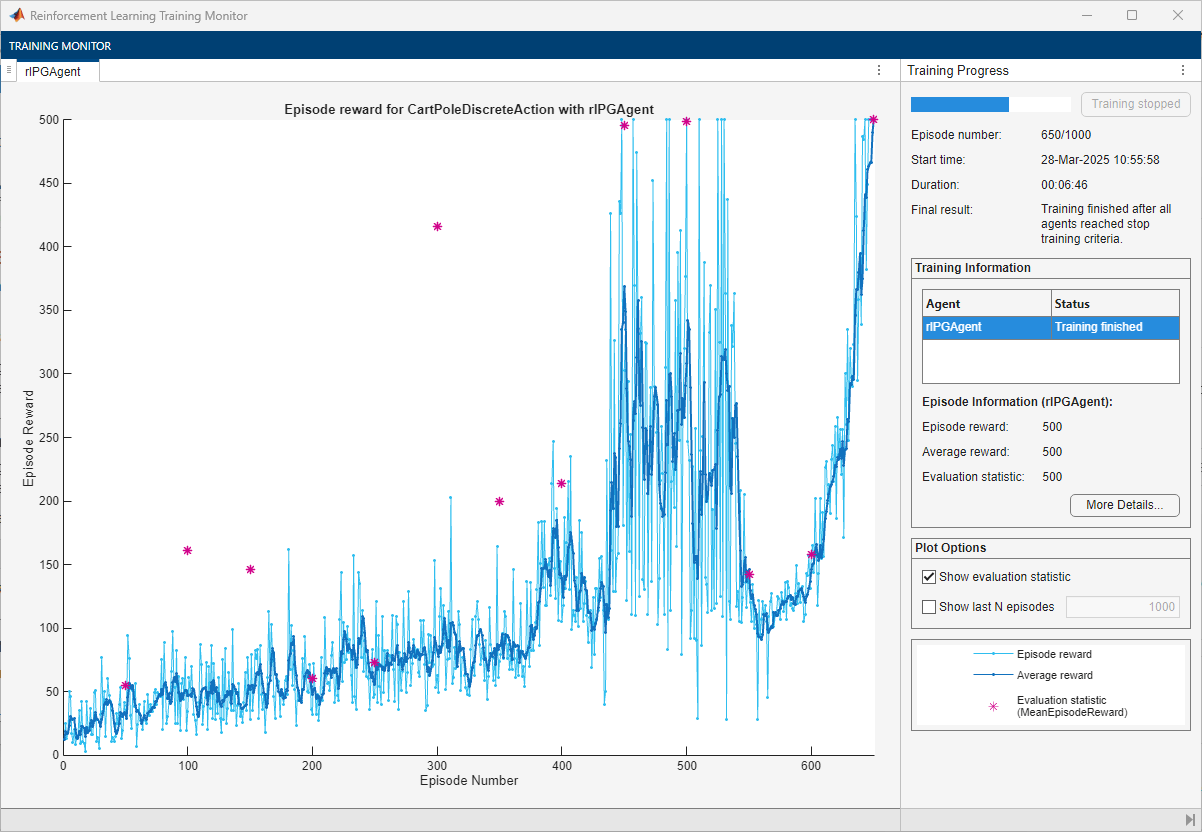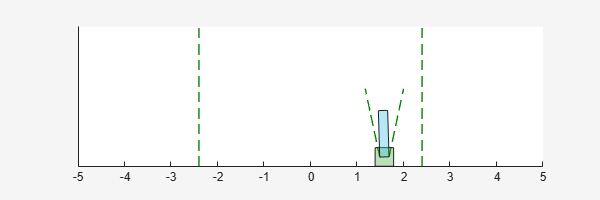# Train PG Agent to Balance Cart-Pole System

This example shows how to train a policy gradient (PG) agent to balance a cart-pole system modeled in MATLAB®. For more information on PG agents, see Policy Gradient (PG) Agents.

For an example that trains a PG agent with a baseline, see Train PG Agent with Baseline to Control Double Integrator System.

### Cart-Pole MATLAB Environment

The reinforcement learning environment for this example is a pole attached to an unactuated joint on a cart, which moves along a frictionless track. The training goal is to make the pendulum stand upright without falling over.For this environment:

• The upward balanced pendulum position is `0` radians, and the downward hanging position is `pi` radians.

• The pendulum starts upright with an initial angle between –0.05 and 0.05 radians.

• The force action signal from the agent to the environment is either –10 or 10 N.

• The observations from the environment are the position and velocity of the cart, the pendulum angle, and the pendulum angle derivative.

• The episode terminates if the pole is more than 12 degrees from vertical or if the cart moves more than 2.4 m from the original position.

• A reward of +1 is provided for every time step that the pole remains upright. A penalty of –5 is applied when the pendulum falls.

### Create Environment Interface

Create a predefined environment interface for the pendulum.

`env = rlPredefinedEnv("CartPole-Discrete")`
```env = CartPoleDiscreteAction with properties: Gravity: 9.8000 MassCart: 1 MassPole: 0.1000 Length: 0.5000 MaxForce: 10 Ts: 0.0200 ThetaThresholdRadians: 0.2094 XThreshold: 2.4000 RewardForNotFalling: 1 PenaltyForFalling: -5 State: [4x1 double] ```

The interface has a discrete action space where the agent can apply one of two possible force values to the cart, –10 or 10 N.

Obtain the observation and action information from the environment interface.

```obsInfo = getObservationInfo(env); actInfo = getActionInfo(env);```

Fix the random generator seed for reproducibility.

`rng(0)`

### Create PG Agent

For policy gradient agents, the actor executes a stochastic policy, which for discrete action spaces is approximated by a discrete categorical actor. This actor must take the observation signal as input and return a probability for each action.

To approximate the policy within the actor, use a deep neural network. Define the network as an array of layer objects, and get the dimension of the observation space and the number of possible actions from the environment specification objects. For more information on creating a deep neural network policy representation, see Create Policies and Value Functions.

```actorNet = [ featureInputLayer(prod(obsInfo.Dimension)) fullyConnectedLayer(10) reluLayer fullyConnectedLayer(numel(actInfo.Elements)) softmaxLayer ];```

Convert to `dlnetwork` and display the number of weights.

```actorNet = dlnetwork(actorNet); summary(actorNet)```
``` Initialized: true Number of learnables: 72 Inputs: 1 'input' 4 features ```

Create the actor representation using the specified deep neural network and the environment specification objects. For more information, see `rlDiscreteCategoricalActor`.

`actor = rlDiscreteCategoricalActor(actorNet,obsInfo,actInfo);`

To return the probability distribution of the possible actions as a function of a random observation, and given the current network weights, use `evaluate`.

```prb = evaluate(actor,{rand(obsInfo.Dimension)}); prb{1}```
```ans = 2x1 single column vector 0.7229 0.2771 ```

Create the agent using the actor. For more information, see `rlPGAgent`.

`agent = rlPGAgent(actor);`

Check the agent with a random observation input.

`getAction(agent,{rand(obsInfo.Dimension)})`
```ans = 1x1 cell array {[-10]} ```

Specify training options for the actor. Alternatively, you can use `rlPGAgentOptions` and `rlOptimizerOptions` objects.

```agent.AgentOptions.CriticOptimizerOptions.LearnRate = 5e-3; agent.AgentOptions.CriticOptimizerOptions.GradientThreshold = 1;```

### Train Agent

To train the agent, first specify the training options. For this example, use the following options.

• Run each training episode for at most 1000 episodes, with each episode lasting at most 500 time steps.

• Display the training progress in the Episode Manager dialog box (set the `Plots` option) and disable the command line display (set the `Verbose` option to `false`).

• Stop training when the agent receives an average cumulative reward greater than 480 over 100 consecutive episodes. At this point, the agent can balance the pendulum in the upright position.

For more information, see `rlTrainingOptions`.

```trainOpts = rlTrainingOptions(... MaxEpisodes=1000, ... MaxStepsPerEpisode=500, ... Verbose=false, ... Plots="training-progress",... StopTrainingCriteria="AverageReward",... StopTrainingValue=480,... ScoreAveragingWindowLength=100);```

You can visualize the cart-pole system by using the `plot` function during training or simulation.

`plot(env)`Train the agent using the `train` function. Training this agent is a computationally intensive process that takes several minutes to complete. To save time while running this example, load a pretrained agent by setting `doTraining` to `false`. To train the agent yourself, set `doTraining` to `true`.

```doTraining = false; if doTraining % Train the agent. trainingStats = train(agent,env,trainOpts); else % Load the pretrained agent for the example. load("MATLABCartpolePG.mat","agent"); end```### Simulate PG Agent

To validate the performance of the trained agent, simulate it within the cart-pole environment. For more information on agent simulation, see `rlSimulationOptions` and `sim`. The agent can balance the cart-pole system even when the simulation time increases to 500 steps.

```simOptions = rlSimulationOptions(MaxSteps=500); experience = sim(env,agent,simOptions);````totalReward = sum(experience.Reward)`
```totalReward = 500 ```